Tuesday , March 19 2019
Recent Post
Home / Quantitative Aptitude Tricks / Profit & Loss Shortcut Tricks Tips & Concept

# Profit & Loss Shortcut Tricks Tips & Concept

 150+ RRB NTPC Previous Solved Papers PDF Free Download Now RRB NTPC Free Study Material PDF Free Download NowRRB NTPC Free Mock TestAttempt It Now Quant Booster eBook – A Complete Maths Shortcut eBook GET Book Now SSC CGL,CPO,GD 2018 Study Material & Book Free PDF Free Download Now

# Profit & Loss Tricks & Tips

#### Profit and Loss questions are important from both examination point of view as well as in different,life situations. Different competitive exams include 1 or2 questions.

These formulas and shortcuts will be helpful for your upcoming Exams like SSC CGL,CHSL & MTS 2017 Exams. Asked questions are based on per cent Profit/Loss. cost price, selling price, price after increase or decrease in rates, cost price of certain number of things equal to S.P. of certain number of, how much price to increase to get certain profit

Cost Price:-
Cost Price is the price at which an article is purchased, abbreviated as C.P

Selling Price:-
Selling Price is the price at which an article is sold, abbreviated as S.P.

Profit:-
If the Selling Price exceeds the Cost Price, then there is Profit.

Important Formulas
Profit –> SP> CP

Profit = SP – CP

Loss –> CP> SP

Loss = CP – SP

Profit% or Gain % –> Profit /CP ×100% = SP – CP / CP ×100%

Loss% –> Loss/CP ×100% = CP – SP / CP×100%

1. One can generate a profit only if Selling Price> Cost Price

2. One generates a loss when Selling Price < Cost Price.

3. Profit = Selling Price – Cost Price
%profit = {(Selling Price – Cost Price)/Cost Price} x 100

4. Loss = Cost Price – Selling Price
%Loss = {(Cost Price – Selling Price)/Cost Price} x 100

5. Sale price :- If there is a profit of P %,
Cost Price = C
Then SP = {(100+P)/100}xC

6. If there is a loss of L %,
Cost Price = C
Then
SP = {(100-L)/100}xC

7. Cost price :-
If there is a profit of P %,
Cost Price = C
Sale price= SP
Then C = {100/(100+p)} x SP
If there is a loss of L %,
Then
C = {100/(100-L)}xSP

8. A dishonest dealer claims to sell his goods at cost price ,but he uses a weight of lesser weight .Find his gain%.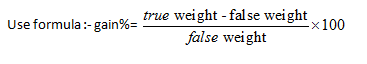9. A shopkeeper sells an item at a profit of x % and uses a weight which is y % less .find his total profit10. When dealer sells goods at loss on cost price but uses less weight .11. A dishonest dealer sells goods at x % loss on cost price but uses a gm instead of b gm . his profit or loss percent :-Note :- profit or loss will be decided according to sign .if +ive it is profit ,if –ve it is loss .

12.  If the price of an item increases by r%  , then the reduction in consumption so that  expenditure remains the same  ,is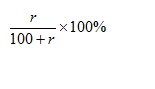13. If the price of a commodity decreases by r% then increase in consumption , so as not to decrease expenditure on this item is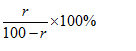14. A reduction of x% in price enables a person to buy y kg more for Rs. A. Then the15. When there are two successive profits of x% and y% then the net percentage profit =[x+y+xy/100]

When there is a profit of x% and loss of y% then net percentage profit or loss = [x – y – xy/100]

Note: If the final sign in the above expression is positive then there is net profit but if it is negative then there is net loss.

16. A sells goods to B at a profit of x% andB sells it to C at a profit of y%. If C pays RsP for it,then the cost price for A is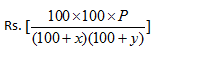Note:- for loss replace plus sign with minus .

17. When each of the two things is sold at the same price,and a profit of p% is made on the first and a loss of L% is made on the second,then the percentage gain or loss is .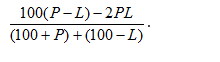18. If profit percentage and loss percentage are equal, put P=L

=>   %loss = p2 /100

19.Type 1:
If two articles are sold- first article at x% profit and the second article at y% loss, thus making no profit, no loss in the transaction,Then cost price of the two articles are in the ratio y : x

Example:
1) A man purchased two articles for total cost of Rs.9000. He sold the first article at 15% profit and the second at 12% loss. In the bargain, he neither gained nor  lost anything. Find cost price of the first article.

Solution:
Ratio of cost price of the first and the second article = 12 : 15 = 4: 5
Cost price of the first article = 4/9 ×9000 = Rs.4000

For Example:

(Q1)A man buys a cycle for Rs. 1400 and sells it at a loss of 15%. What is the selling price of the cycle?

(a)Rs 1202 (b)Rs 1190 (c)Rs 1160 (d)Rs 1000

Solution: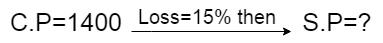=>1400×(100-15)/100=S.P

=>1400×(85/100)=S.P

S.P=Rs. 1190 Ans.

(Q2)A man buys a cycle for Rs. 1400 and sells it at a profit of 20%. What is the selling price of the cycle?

(a)Rs 1500 (b)Rs 1560 (c)Rs 1580 (d)Rs 1680

Solution: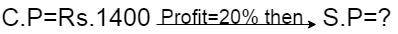=>1400×(100+20)/100=S.P

=>1400×(120/100)=S.P

S.P=Rs. 1680 Ans.

(Q3)There is 10% loss if an article is sold at Rs. 270, then the cost price of the article is.

(a)Rs 300 (b)Rs 270 (c)Rs 320 (d)Rs 250

Solution: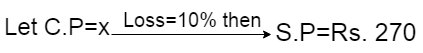=>x×(100-10)/100=270

=>x×(90/100)=270

∴ x=(270×100)/90

=Rs. 300 Ans.

BY Formula:

C.P=(S.P×100)/(100-Loss%)

C.P=(270×100)/(100-10)

=(270×100)/90

=Rs. 300 Ans.

(Q4)An item when sold for Rs. 1690 earned 30% profit on the cost price. Then the cost price is.

(a)Rs 507 (b)Rs 630 (c)Rs 1300 (d)Rs 130

Solution: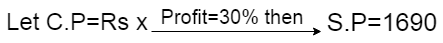=>x×(100+30)/100=1690

=>x×(130/100)=1690

∴ x=(1690×100)/130

=Rs. 1300 Ans.

BY Formula:

C.P=(S.P×100)/(100+Profit%%)

C.P=(1690×100)/(100+30)

=(1690×100)/130

=Rs. 1300 Ans.

(Q5)A man buys an article for Rs. 27.50 and sells it for Rs. 28.60.Find his gain percent.

(a)5% (b)6% (c)4% (d)3%

Solution:C.P=Rs. 27.50

S.P=Rs. 28.60

Gain=S.P-C.P

=Rs. 28.60-Rs. 27.50

=Rs. 1.10

∴ Gain%= (Profit or Gain×100)/C.P

= (1.10×100)/27.50

= 4% Ans.

(Q6)If a radio is purchased for Rs. 490 and sold for Rs. 465.50, find the loss percent.

(a)5% (b)6% (c)4% (d)3%

Solution:C.P=Rs. 490

S.P=Rs. 465.50

Loss=C.P-S.P

=Rs. 490-Rs. 465.50

=Rs. 24.50

∴ Loss%=(Loss×100)/C.P

=(24.50×100)/490

=5% Ans.

(Q7)A fan is listed at Rs. 150 and a discount of 20% are given. Then the selling price is.

(a)Rs 180 (b)Rs 150 (c)Rs 120 (d)Rs 110

Solution:M.P=Rs. 150 Discount%=20%

Discount=150×(20/100)

=Rs. 30

S.P=M.P-Discount

=Rs. 150-Rs. 30

=Rs 120 Ans.

Another way:=>150×(100-20)/100=S.P

=>150×(80/100)=S.P

S.P=Rs 120 Ans.

(Q8)If a Book costs Rs. 64 after 20% discount is allowed. What was its original price(i.e M.P) in Rs. ?

(a)Rs 80 (b)Rs 50 (c)Rs 75 (d)Rs 85

Solution: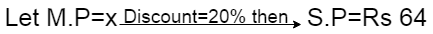=>x×(100-20)/100=64

=>x×(80/100)=64

∴ x=(64×100)/80

= Rs 80 Ans.

(Q9)A merchant purchases a table for Rs 450 and fixes its list price in such a way that after allowing a discount of 10%, he earns a profit of 20%. Then the list price of the table is.

(a)Rs 650 (b)Rs 700 (c)Rs 550 (d)Rs 600

Solution:C.P=Rs 450

Discount=10% and Profit=20%

M.P=?=>450×(100+20)/100=S.P

=>450×(120/100)=S.P

S.P=Rs 540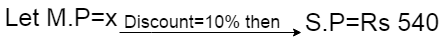=>x×(100-10)/100=540

=>x×(90/100)=540

∴ x=(540×100)/90

=Rs 600 Ans.

(Q10)Mohan purchased a machine for Rs 80,000 and spent Rs 5000 on repair and Rs 1000 on transport and sold it with 25% profit. At what price did he sell the machine?

(a)Rs 107500 (b)Rs 105700 (c)Rs 107550 (d)Rs 117600

Solution:Total cost price=80,000+5000+1000

=Rs 86,000

S.P=86000×(100+25)/100

=86000×(125)/100

=Rs 107500 Ans.

(Q11)An Egg seller buys 240 egg for Rs. 600.Some of these eggs are bad and are thrown away.He sells the remaining egg at Rs. 3.50 each and makes a profit of 198. The percent of an egg thrown away are.

(a)6% (b)8% (c)5% (d)7%

Solution:C.P of an egg=600/240

=Rs. 2.5

profit=Rs 198

Total S.P=Profit+C.P     [where Profit or Gain= S.P-C.P ]

= 198+600=Rs 798

Number of egg sold=798/3.5

=228

Now,

Required percentage=(12×100)/240

= 5% Ans.

(Q12)A shopkeeper bought 30Kg of rice at the rate of Rs. 70 per kg and 20 Kg of rice at a rate of Rs. 70.75 per Kg. If he mixed the two brands of rice and sold the mixture at Rs. 80.50 per Kg, his gain is.

(a)Rs 450 (b)Rs 510 (c)Rs 525 (d)Rs 485

Solution: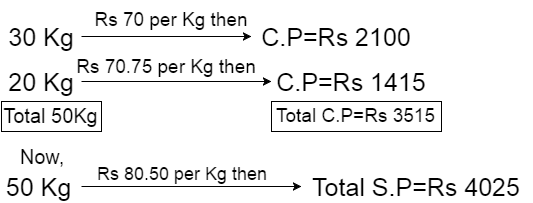Gain=S.P-C.P

= 4025-3515=Rs 510 Ans.

(Q13)A profit of 12% is made when a mobile phone is sold at Rs P and there is 4% loss when the phone is sold at Rs Q. Then Q:P is.

(a)1:1 (b)4:5 (c)6:7 (d)3:1

Solution:=>x×(100+12)/100=P

=>x×(112/100)=P

112x=100P

P=112x/100

Now,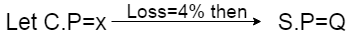=>x×(100-4)/100=Q

=>x×(96/100)=Q

=>96x=100Q

Q=96x/100

Now,

Q/P=(96x/100)/(112x/100)

=6/7

Q:P=6:7 Ans.

(Q14)If the ratio of cost price to selling price is 10:11, Then the rate of a percent of profit is.

(a)1.1% (b)0.1% (c)10% (d)1%

Solution:Let C.P=10x

S.P=11x

Profit=11x-10x=x

∴ % Profit=(x×100)/10x

=10% Ans.

(Q15)An article is sold at 5% profit. The ratio of selling price and cost price will be.

(a)1:5 (b)20:21 (c)21:20 (d)5:1

Solution:=>x×(100+5)/100=S.P

S.P=105x/100

Now,

S.P/C.P=(105x/100)/x

=21/20

∴ S.P:C.P=21:20 Ans.

(16)A store sells watch for a profit of 25% of the cost. Then the percentage of profit against selling price is.

(a)22% (b)20% (c)18% (d)15%
Solution:-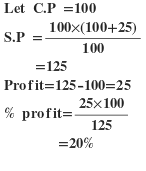Second Method:-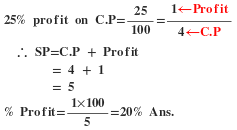(17)By selling an article, a man makes a profit of 25% of its selling price. His profit percent is.
(a)20% (b)25% (c)16 ²/3 %  (d)33 ¹/3 %

Solution:-(18)If the cost price is 90% of the selling price, what is the profit percent?
(a)4% (b)4.75% (c)5% (d)11 ¹/3 %
Solution:-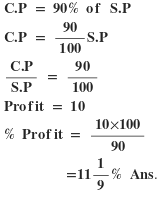For Example:

(Q1)If a man were to sell his chair for Rs. 720, he would loss 25%.To gain 25% he would sell it for.

Solution:

Step-1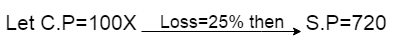100x×(100-25)/100=720

75x=720

∴ x=720/75

C.P=100x=100×(720/75)=Rs. 960

Step-2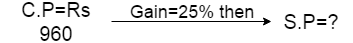960×(100+25)/100=S.P

960×(125/100)=S.P

S.P=Rs. 1200 Ans.

BY TRICK:

Desire Gain(D Gain)=25%

Loss=25%

S.P=Rs(720×(100+D Gain))/(100-loss)

=Rs(720×125)/75

=Rs. 1200 Ans.

(Q2)By selling an article for Rs 600 a man gains 20%. At what price should he have sold it to gain 15%?

Solution:

Step-1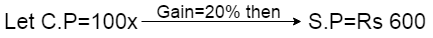100x×(100+20)/100=Rs 600

120x=Rs 600

x=5

∴ C.P=100x=100×5

=Rs 500

Step-2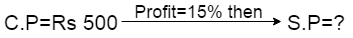500×(100+15)/100=S.P

S.P=Rs 575 Ans.

BY TRICK:

Desire Gain(D Gain)=15%

Normal Gain=20%

S.P=Rs(600×(100+D Gain))/(100+Gain)

=Rs(600×115)/120

=Rs. 575 Ans.

(Q3)By selling an article for Rs 720 a man loss 20%. At what price should he have sold it to loss 15%?

Solution:

Step-1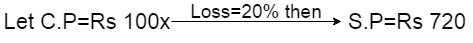100x×(100-20)/100=720

80x=720

∴ x=720/80=9

C.P=100x=100×9=Rs 900

Step-2900×(100-15)/100=S.P

900×(85/100)=S.P

S.P=Rs 765 Ans.

BY TRICK:

Desire Loss(D Loss)=15%

Loss=20%

S.P=Rs(720×(100-D Loss))/(100-Loss)

=Rs(720×85)/80

=Rs 765 Ans.

(Q4)By selling 80 ball pens for Rs 140 a retailer loses 30%. How many ball pens should he sell for Rs 104 so as to make a profit of 30%?

Solution:

Step-1: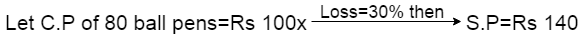100x×(100-30)/100=140

70x=140

x=2

∴ C.P=100x=100×2=Rs 200

Step-2:200×(100+30)/100=S.P

S.P=Rs 260

Now,

Rs 260=80 ball pen

∴ Rs 104=(80/260)×104

=32 ball pens Ans

BY TRICK:

Desire Profit(D-Profit)=30%

Loss=30%

S.P=Rs(140×(100+D Gain))/(100-loss)

=Rs(140×130)/70

=Rs. 260

Now,

Rs 260=80 ball pen

∴ Rs 104=(80/260)×104

=32 ball pens Ans

(Q1)A man sells a car to his friend at 10% loss. If the friend sells it for Rs 54,000 and gains 20% the original cost price of the car was.

Solution: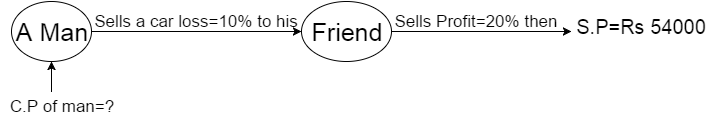Let Original Cost Price or C.P of a Man=100x

100x×((100-10)/100)×(100+20)/100=54000

=>100x×(90/100)×120/100=54000

⇒108x=54000

∴ x=500

Original C.P=100x=100×500

=Rs 50000 Ans.

(Q2)A sold an article to B at the profit of 25%.B sold it to C loss of 10% and C sold it to D at the profit of 20%.If D paid Rs 27 then how much A paid to buy the article.

Solution: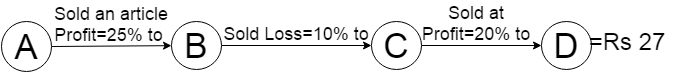Let C.P of A=100x

100x×(125/100)×(90/100)×(120/100)=Rs 27

∴ x=(27×100×100×100)/(100×125×90×120)

=0.2

C.P of A=100x=100×0.2

=Rs 20 Ans.

(Q3)A sold a horse to B for Rs 4800 by losing 20%.B sells it to C at a price which would have given A a profit of 15% B’s gain is.

Solution: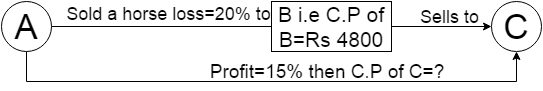Let C.P of A=100x

100x×(80/100)=4800

80x=4800

∴ x=60

C.P of A=100x=100×60

=Rs 6000

Now,

6000×(115/100)=S.P of A

S.P of A(i.e C.P Of C)=6900

Gain of B=6900-4800

=Rs 2100 Ans.

For Example:

(Q1)A vendor bought bananas at 6 for Rs 10 and sold them at 4 for Rs 6.Find his gain or loss percent.

Solution: By Concept And Shortcut Tricks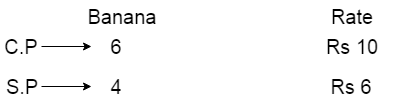Step-2:C.P=40 and S.P=36

Loss=C.P-S.P=40-36=Rs 4

% Loss=(4×100)/40

=10% Ans.

CONCEPT:[इस Type के Problem को solve करते Time Object को Only Equal बनाना है| एवं Object को equal बनाने के लिए जिस Number से Multiply किया जायेगा उसी Number से Rate वाले part को Multiply करना है]

Note: Above वाले method से आप लोग इस तरह के problem को 10 sec में solve कर सकते है और Formula याद रखने के कोई जरूरत नहीं है|

(Q2)A man buys lemons at the rate of 3 for Rs 5 and sells them at 2 for Rs 4 what is the profit percent?

Solution: By Concept And Shortcut TricksC.P=Rs 10 and S.P=Rs 12

Profit=S.P-C.P

=Rs(12-10)=Rs 2

% profit=(2×100)/10

=20% Ans.

(Q3)A man purchases some oranges at a rate of 3 for Rs 40 and same quantity at 5 for Rs 60. If he sells all the oranges at the rate of 3 for Rs 50, find his gain or loss percentage.

Solution: By Concept And Shortcut Tricks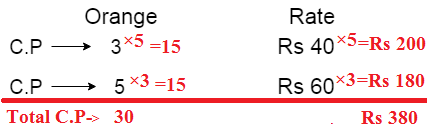Now,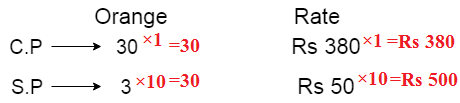C.P=Rs 380 And S.P=Rs 500

Profit=S.P-C.P

=Rs(500-380)

=Rs 120

% profit=(120×100)/380

=32% Ans.

(Q1)The cost price of 15 articles is same as the selling price of 10 articles. The profit percent is.

Solution: By Concept And Shortcut Tricks

C.P of 15 articles = S.P of 10 articles

C.P/S.P=10/15

Profit=S.P-C.P

=15-10=5 articles

∴ Profit %=(5×100)/10

=50% Ans.

By Tricks:=((15-10)/10)×100

=(5/10)×100

=50% Ans.

(Q2)The selling price of 12 objects is equal to the cost price of 9 objects. Find the Loss or Profit percentage?

Solution: By Concept And Shortcut Tricks

S.P of 12 objects =C.P of 9 objects

S.P/C.P=9/12

Note:C.P>S.P so loss

Loss=C.P-S.P

=12-9=3

%Loss=(3×100)/12

=25% Ans.

By Tricks:=((12-9)/12)×100

=(3/12)×100

=25% Ans.

(Q3)By selling 33m cloth a shopkeeper earns profit equivalent to selling price of 11m. Find his profit percent.

Solution: By Concept And Shortcut Tricks

Profit     =    S.P-C.P

S.P(11m)=s.p(33m)-C.P(33m)

⇒C.P(33m)=s.p(33m)-S.P(11m)

⇒C.P(33m)=s.p(22m)

⇒C.P/S.P=22m/33m

Profit=33m-22m=11m

% Profit=(11×100)/22

=50% Ans

By Tricks:

% Profit=(Profit/S.P-Profit)×100

=(11/33-11)×100

=(11/22)×100

=50% Ans.

(Q4)By selling 33m, a shopkeeper incurs loss equivalent to the cost price of 11m. Find his loss percent.

Solution: By Concept And Shortcut Tricks

Loss     = C.P-S.P

C.P(11m)=C.P(33m)-S.P(33m)

⇒S.P(33m)=C.P(33m)-C.P(11m)

⇒S.P(33m)=C.P(22m)

⇒S.P/C.P=22m/33m

Loss=(33-22)m=11m

%Loss=(11×100)/33

=33 ¹/3% Ans.

If two article are sold at equal prices, the First one is sold at X% profit whereas the second one at a loss of X% then the seller always incurs a loss in the entire Transition.

It is equal to ( X)²/100

(Q1) A dealer sold two T.V sets for Rs 7400 each on one he gained 10% and on the other, he lost 10%. The dealer’s loss or gain in the transaction is.

Solution:

% Loss=( X)²/100

=( 10)²/100

=100/100=1% Ans.

(Q2) a dealer sold two T.V sets for Rs 990 each on one he gained 10% and on the other he lost 10%. Then

(1)Find total C.P of T.V.

(2)His loss on the whole in Rs is.

Solution:

% Loss=( X)²/100

=( 10)²/100

=100/100=1%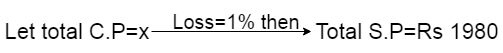x×(100-1)/100=1980

x×99/100=1980

∴ x=(1980×100)/99

=Rs 2000 Ans.

(2)Loss=C.P-S.P

=Rs(2000-1980)

=Rs 20 Ans.

Trick: If two article is bought at equal prices, the First one is sold at X% profit whereas the second one at a loss of X% then the seller always incurs a no loss and no profit in the entire Transition.

(Q1)A dealer bought two T.V sets for Rs 7400 each. The First one is sold at 10% profit whereas the second one at a loss of 10%. Then find out loss or gain in the transaction.

Solution: No Loss And No Profit.

Note:[We know that If two article is bought at an equal price, the First one is sold at X% profit whereas the second one at a loss of X% then the seller always incurs a no loss and no profit in the entire Transition.]

Trick: If two article are sold at equal prices, the First one is sold at P% profit whereas the second one at a loss of L% then theNote: If +ve then profit or if -ve then loss

(Q1) A man sold two watches for Rs 525 each. On one he gains 10% and on the other he loses 15%. His profit or loss in the whole transaction is.

Solution:=((100×-5)-2×10×15)/110+85

=(-500-300)/195

=-4.10% ie Loss=4.10% Ans.

By Concept: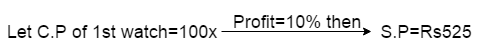100x×(100+10)/100=525

⇒ (100x×110)/100=525

∴ x=525/110

C.P of 1st watch=100x

=100×525/110

=Rs 477.27

Now,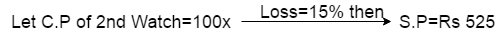100x×(100-15)/100=525

⇒ (100x×85)/100=525

∴ x=525/85=6.17

C.P of 2nd watch=100x

=100×6.17

=Rs 617

Now,

Total C.P=Rs(477.27+617)

=Rs 1094.27=1094

Total S.P=525+525=Rs 1050

Loss=C.P-S.P

=Rs(1094-1050)

=Rs 48

% Loss=(48×100)1094

=4.10% Ans.

 GovernmentAdda RecommendsOnline Tyari Mock Test RRB Group D Speed Test Attempt NowAttempt Now Railway RRB Pshyco Complete PackageGet It Now SSC CHSL, CGL Mock Test (1 free+30 Paid)Attempt Now